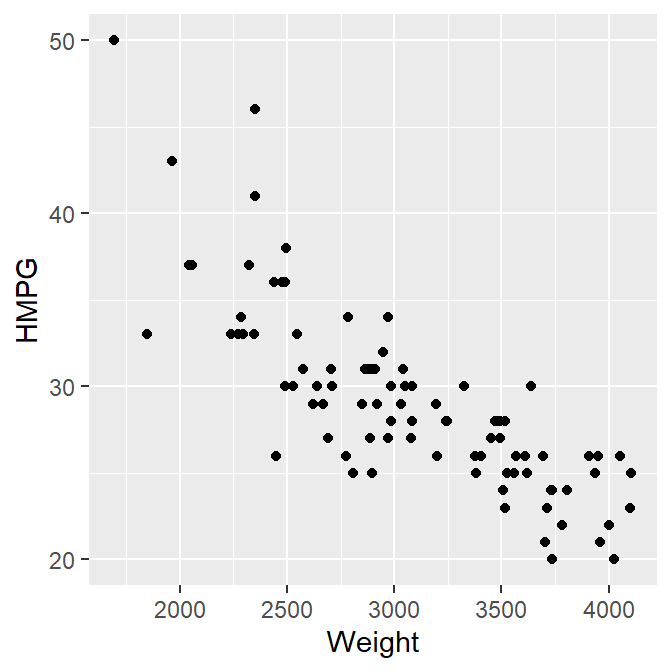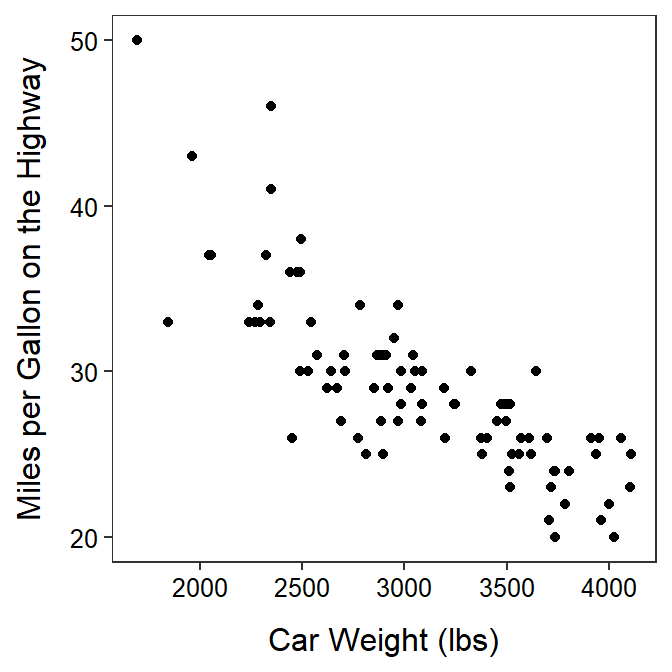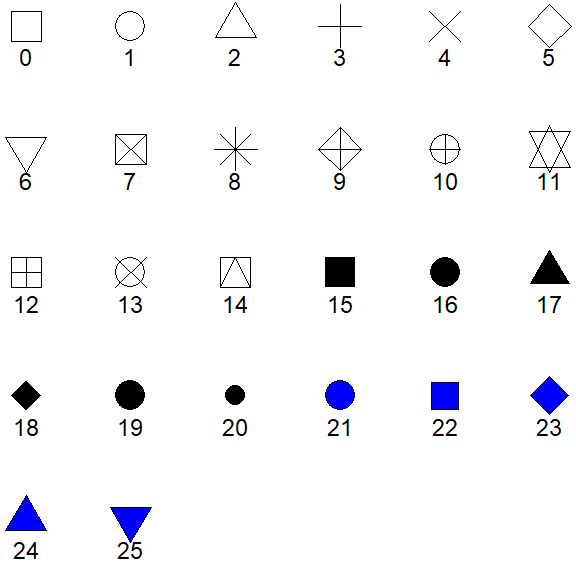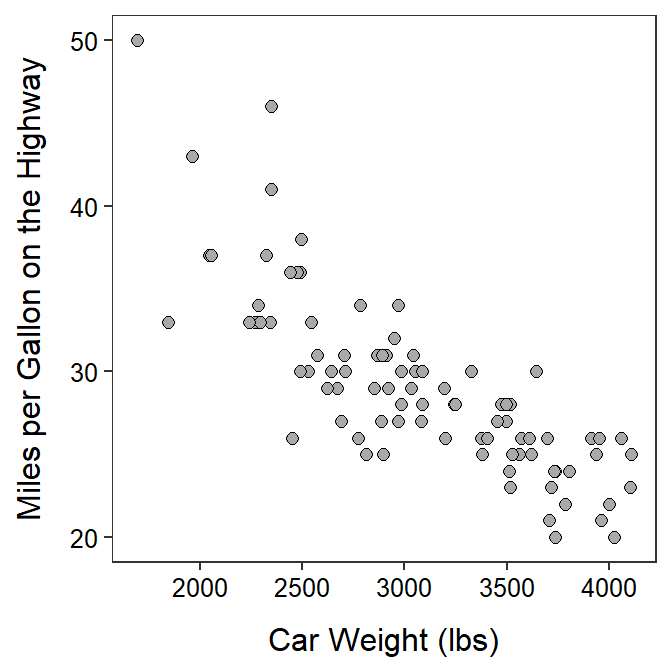# Module 26 Bivariate EDA in R

In the Modules 8 and 10 you practiced performing an EDA for pairs of quantitative or categorical data using summary statistics and graphics. In this module, you will learn how to construct those summary statistics and graphics from data using R.96 You will also be asked to perform the EDA from these results.

#### Data Sets

The quantitative summaries and graphics will use the weight (lbs) and highway miles per gallon (HMPG) for 93 cars from the 1993 model year. Ultimately the relationship between highway MPG and the weight of a car is described. These data are in 93cars.csv (data, meta) and are loaded into R below with the methods described in Section 23.1.2.97

cars93 <- read.csv("93cars.csv")
headtail(cars93,which=c("MFG","Model","Type","Weight","HMPG"))
#R>            MFG   Model    Type Weight HMPG
#R>  1       Acura Integra   Small   2705   31
#R>  2       Acura  Legend Midsize   3560   25
#R>  3        Audi      90 Compact   3375   26
#R>  91 Volkswagen Corrado  Sporty   2810   25
#R>  92      Volvo     240 Compact   2985   28
#R>  93      Volvo     850 Midsize   3245   28

Methods for categorical data will be demonstrated with two questions from the General Sociological Survey (GSS).

• What is your highest degree earned? [choices – “less than high school diploma,” “high school diploma,” “junior college,” “bachelors,” or “graduate”; labeled as degree]
• How willing would you be to accept cuts in your standard of living in order to protect the environment? [choices – “very willing,” “fairly willing,” “neither willing nor unwilling,” “not very willing,” or “not at all willing”; labeled as grnsol]

These data, stored in GSSWill2Pay.csv (data,meta), are loaded into R and examined below.

gss <- read.csv("GSSWill2Pay.csv")
str(gss)
#R>  'data.frame':  3955 obs. of  2 variables:
#R>   $degree: chr "ltHS" "ltHS" "ltHS" "ltHS" ... #R>$ grnsol: chr  "vwill" "vwill" "vwill" "vwill" ...

As is typical, the categorical variables are recorded as character type. Both degree and grnsol are ordinal categorical variables and, thus, should be converted to factor variables types so that the order of the levels can be controlled. To assist with this, use unique() to see the exact spelling of each of the levels.

unique(gss$degree) #R>  "ltHS" "HS" "JC" "BS" "grad" unique(gss$grnsol)
#R>   "vwill"   "will"    "neither" "un"      "vun"

The variables are then converted to factors below with factor() using levels= to control the order of the variables.98

gss$degree <- factor(gss$degree,levels=c("ltHS","HS","JC","BS","grad"))
gss$grnsol <- factor(gss$grnsol,levels=c("vwill","will","neither","un","vun"))

The levels are checked with levels() below to make sure that the order is correct and that none are missing (which would imply that the level was mis-spelled above).

levels(gss$degree) #R>  "ltHS" "HS" "JC" "BS" "grad" levels(gss$grnsol)
#R>   "vwill"   "will"    "neither" "un"      "vun"

Ordinal variables should be converted to factor variables in R, with the levels controlled to their natural (rather than alphabetical) order.

## 26.1 Quantitative

### 26.1.1 Scatterplots

Scatterplots are constructed by giving the x- and y-axis variables to x= and y= in aes() in ggplot()99 and then adding geom_point().

ggplot(data=cars93,mapping=aes(x=Weight,y=HMPG)) +
geom_point()Define both x and y variables for a scatterplot.

The axis labels can be properly labeled with labs() and the theme used in the course applied with theme_NCStats().100

ggplot(data=cars93,mapping=aes(x=Weight,y=HMPG)) +
geom_point() +
labs(x="Car Weight (lbs)",y="Miles per Gallon on the Highway") +
theme_NCStats()There may be times where you want to change the shape of symbol that is plotted by including a numeric code to shape= in geom_point(). Shape codes are shown in Figure 26.1.Figure 26.1: Point shapes available in R and their numerical codes. For shapes 0-20 that color= controls the shapes color, but for shapes 21-25 color= controls the outline color and fill controls the inside color of the shape.

My typical choice for plotting points are shown below.101

ggplot(data=cars93,mapping=aes(x=Weight,y=HMPG)) +
geom_point(shape=21,color="black",fill="darkgray",size=2) +
labs(x="Car Weight (lbs)",y="Miles per Gallon on the Highway") +
theme_NCStats()When making your own scatterplot, copy the code above and change the items in data=, x=, y=, x=, and y=. All other items can remain as shown above.

### 26.1.2 Correlation Coefficient

The correlation coefficient (r) between two quantitative variables is computed with corr() using a formula of the form ~qvarY+qvarX,102 where qvarY and qvarX are the names of quantitative variables, as the first argument and the corresponding data frame in data=.103 The number of decimal places is controlled with digits=.104 For example, the correlation coefficient between highway MPG and weight for all cars in the car data is -0.811.

corr(~HMPG+Weight,data=cars93,digits=3)
#R>   -0.811
corr(HMPG~Weight,data=cars93,digits=3)   # alternative form
#R>   -0.811

The correlation coefficient can be computed for pairs of multiple variables by “adding” the multiple variables to the formula described above. Note that use="pairwise.complete.obs" should be used used to make sure that missing data is removed when calculating the correlation coefficient for each pair of variables.

corr(~HMPG+FuelTank+Length+Weight,data=cars93,use="pairwise.complete.obs",digits=3)
#R>             HMPG FuelTank Length Weight
#R>  HMPG      1.000   -0.786 -0.543 -0.811
#R>  FuelTank -0.786    1.000  0.690  0.894
#R>  Length   -0.543    0.690  1.000  0.806
#R>  Weight   -0.811    0.894  0.806  1.000

## 26.2 Categorical

### 26.2.1 Frequency Tables

Two-way frequency tables are constructed in R with xtabs(), where the first argument is a formula of the form ~cvarRow+cvarCol, where cvarRow and cvarCol are categorical variables to form the rows and columns of the table, in the first agument and the corresponding data frame in data=. The result of xtabs() should be assigned to an object for further use.

( tbl1 <- xtabs(~degree+grnsol,data=gss) )
#R>        grnsol
#R>  degree vwill will neither  un vun
#R>    ltHS    40  145     132 151 178
#R>    HS      87  542     512 557 392
#R>    JC      15   61      64  54  44
#R>    BS      42  199     179 187  75
#R>    grad    24  104      83  64  24

Totals may be added to the margins of a saved table with addMargins().

addMargins(tbl1)
#R>        grnsol
#R>  degree vwill will neither   un  vun  Sum
#R>    ltHS    40  145     132  151  178  646
#R>    HS      87  542     512  557  392 2090
#R>    JC      15   61      64   54   44  238
#R>    BS      42  199     179  187   75  682
#R>    grad    24  104      83   64   24  299
#R>    Sum    208 1051     970 1013  713 3955

### 26.2.2 Percentage Tables

Percentage tables are constructed in R by submitting the saved xtabs() object to percTable().105 A total- or table-percentage table is constructed by default.

percTable(tbl1)
#R>        grnsol
#R>  degree vwill will neither   un  vun  Sum
#R>    ltHS   1.0  3.7     3.3  3.8  4.5 16.3
#R>    HS     2.2 13.7    12.9 14.1  9.9 52.8
#R>    JC     0.4  1.5     1.6  1.4  1.1  6.0
#R>    BS     1.1  5.0     4.5  4.7  1.9 17.2
#R>    grad   0.6  2.6     2.1  1.6  0.6  7.5
#R>    Sum    5.3 26.5    24.4 25.6 18.0 99.8

A row-percentage table is constructed by including margin=1 in percTable().

percTable(tbl1,margin=1)
#R>        grnsol
#R>  degree vwill  will neither    un   vun   Sum
#R>    ltHS   6.2  22.4    20.4  23.4  27.6 100.0
#R>    HS     4.2  25.9    24.5  26.7  18.8 100.1
#R>    JC     6.3  25.6    26.9  22.7  18.5 100.0
#R>    BS     6.2  29.2    26.2  27.4  11.0 100.0
#R>    grad   8.0  34.8    27.8  21.4   8.0 100.0

A column-percentage table is constructed by including margin=2 in percTable().

percTable(tbl1,margin=2)
#R>        grnsol
#R>  degree vwill  will neither    un   vun
#R>    ltHS  19.2  13.8    13.6  14.9  25.0
#R>    HS    41.8  51.6    52.8  55.0  55.0
#R>    JC     7.2   5.8     6.6   5.3   6.2
#R>    BS    20.2  18.9    18.5  18.5  10.5
#R>    grad  11.5   9.9     8.6   6.3   3.4
#R>    Sum   99.9 100.0   100.1 100.0 100.1

## 26.3 Generic R Code

The following generic codes were used in this module and are provided here so that you can efficiently copy and paste them into your assignment. Note the following:

• dfobj should be replaced with the name of your data frame.
• qvar1 and qvar2 should be replaced with the names of your quantitative variables.
• cvarRow and cvarCol should be replaced with the names of your categorical variables.
• NUM should be replaced with a number.
• XXX should be replaced with what an individual is.
• better qvar1/qvar2 label should be replaced with descriptive labels for the qvar1 and qvar2 variable.

Also examine the “R Function Guide” on the class Resources page for more guidance.

#### Bivariate EDA Quantitative

• Construct a scatterplot
ggplot(data=dfobj,mapping=aes(y=qvar1,x=qvar2)) +
geom_point(pch=21,color="black",fill="lightgray") +
labs(y="better qvar1 label",x="better qvar2 label") +
theme_NCStats()

• Compute the correlation coefficient
corr(~qvar1+qvar2,data=dfobj,digits=3)

#### Bivariate EDA Categorical

• Construct a frequency table
freq2 <- xtabs(~cvarRow+cvarCol,data=dfobj)
addmargins(freq2)           # append totals

• Construct percentage tables
percTable(freq2)            # total/table %
percTable(freq2,margin=1)   # row %
percTable(freq2,margin=2)   # column %

1. Methods in this module require the NCStats package (as always) and the ggplot2 package (for making graphs). Both packages are loaded in the first code chunk of the assignment template.↩︎

2. The which= argument in headtail() is used to show only a few variables of cars93, just to save space.↩︎

3. Recall from the previous module that these levels must be spelled exactly as they appear in the data frame.↩︎

4. The use of ggplot() was introduced in the previous module.↩︎

5. Both labs() and theme_NCStats() were introduced in the previous module.↩︎

6. The size= is used to make the points larger.↩︎

7. Note that qvarY~qvarX will also work.↩︎

8. corr() is from NCStats.↩︎

9. Two or three decimals should be used for correlation coefficients.↩︎

10. percTable() is from NCStats.↩︎